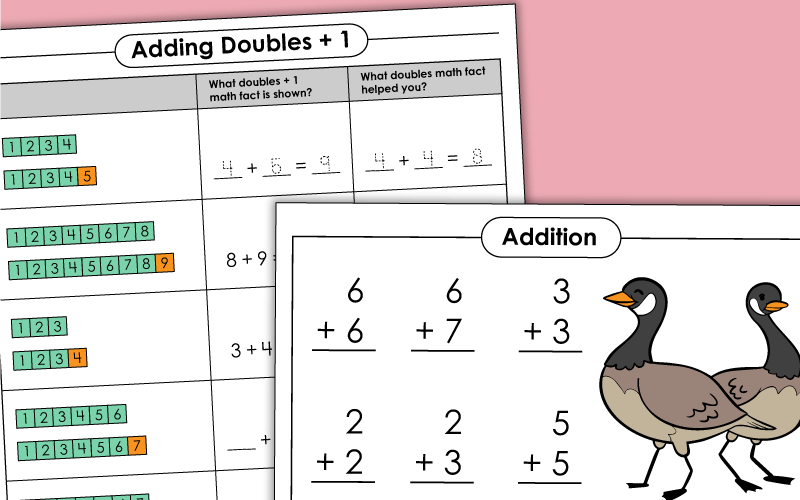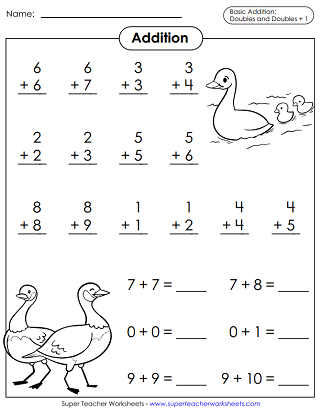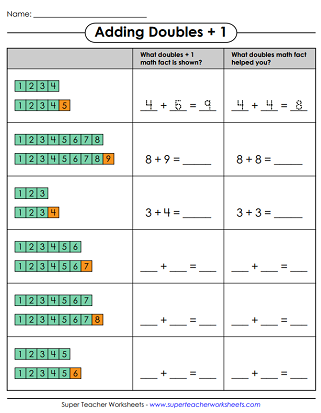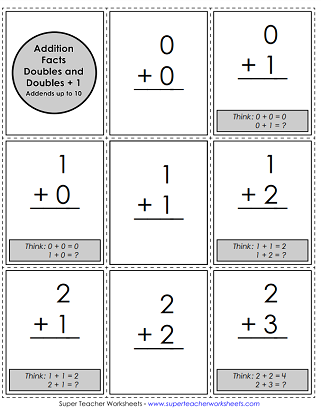# Basic Addition: Doubles Plus 1

Doubles plus 1 is an addition strategy for adding pairs of numbers where an addend is 1 more than the other addend. Students think of a doubles fact and add 1. For example, to solve 3+4, the student thinks of 3+3 (a doubles fact) and then adds 1 to make 7.## Graphical Concept Worksheets: Addition Doubles +1

On this worksheet, number blocks are used as models for basic doubles +1 addition facts.
Use the illustrations of red and white math counters to solve the basic doubles +1 addition facts.
Use illustrations of ten frames with counters to answer the math facts.

## Practice Worksheets

Then sort them into two categories on the t-chart: doubles or doubles +1.
This worksheet helps reinforce both doubles and doubles + 1 math skills. Includes both vertical and horizontal problems.

## Learning Center / Math GameAddition Doubles +1

Flip the cards upside down. Match the addition facts with their sums. The player with the most matches wins. (Recommendation: Print on card stock or thick paper so students cannot see through the cards.)

## Flashcards and Sliders

This file has 31 flash cards for practicing doubles facts and doubles plus 1 facts. There's also a sorting mat on the last page.
Slide the facts through the airplane and say the answers. (Recommendation: Print on card stock for best results.)

Find hundreds of worksheets, games, and printables for teaching basic addition fluency and concepts.

## Sample Worksheet ImagesMy Account
Site Information